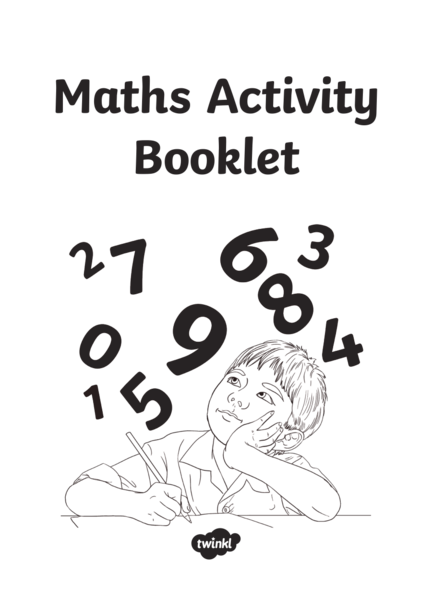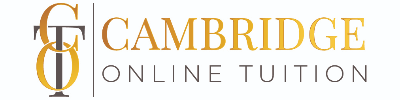# Yr 4 Maths Revision BookletContributed by:1. Maths Activity
Booklet
2. Number and Place Value
1. Continue these number sequences:
9, 18, 27, 36, 45, , , , , , , ,
775, 750, 725, 700, , , , , , , ,
5, 4, 3, 2, , , , , , , ,
2. Find 100 less than these numbers:
3. Find 1000 less than these numbers:
59 003
17 351
20 882
4. What is the value of the underlined digit in each number?
5. Put these numbers in order from smallest to largest.
10 111 11 011 10 011 11 110 11 101
Smallest Largest
6. Compare these numbers using <, > or =.
454 544 660 606 2 tens 4 ones 24 ones
Page 1 of 20
3. Representing Number
1. What number is shown below?
2. Complete the table, showing the numbers in numerals and words.
2109
One thousand, two hundred and
ninety-three.
29 431
Seventy-five thousand and
ninety-eight.
Roman Numeral
3. Use the information in the table to work
out the value of these Roman numerals. I 1
LXXII = V 5
XIV =
X 10
CCLIX=
L 50
C 100
6 7 4. a) What is the largest number that can be made from
these digit cards?
2 b) What is the smallest number that can be made from
these digit cards?
5 9
Page 2 of 20
1. Complete these calculations mentally:
421 + 50 =
376 + 200 =
250 - 99 =
2. Complete these calculations:
1 3 5 7 3 5 9 2 7 9 8 5 5 3 1 9
+ 2 6 4 1 + 4 2 3 8 - 1 3 4 2 - 3 2 6 7
3. Complete these calculations:
3410 + = 5655
6720 – = 5220
4. Use appropriate calculations to solve these problems.
a) At a cinema, there is room for 750 people in a screen. If the cinema sells 641 tickets for a
screen, how many are left?
b) In one day, 2345 people visit the cinema. 1032 of them go and see an action film and the
others go and see a comedy. How many people went to see the comedy?
Page 3 of 20
5. Multiplication and Division
1. Fill in the missing numbers in the multiplication square.
x 1 2 3 4 5 6 7 8 9 10 11 12
1 1 2 4 6 8 9 11 12
2 2 6 8 12 14 18 20 24
3 3 12 15 21 24 30 33
4 8 12 20 24 32 36 44 48
5 5 10 20 25 35 40 50 55
6 6 18 24 30 36 54 60 72
7 14 21 42 49 56 70 77
8 8 16 32 40 56 64 72 88 96
9 18 27 45 54 63 81 90 99 108
10 10 30 40 60 70 80 90 100 120
11 22 33 55 66 88 121
12 12 24 48 60 84 108 120 144
2. Explain the pattern of the 9 times table.
Page 4 of 20
6. Multiplication and Division
3. Complete these calculations:
250 × 4 =
555 × 100 =
2540 × 0 =
4. Use your knowledge of multiplication and division methods to solve these problems.
a) A box of glue sticks contains 128 glue sticks. There are 4 classes in the school. How many
glue sticks does each class get?
b) To make a model, each child needs 8 lolly sticks. If lolly sticks come in packs of 30, how
many packs would be needed for 28 children to make a model?
5. Use formal methods to complete these calculations.
a) 45 × 6 = b) 333 ÷ 9 =
6. If we know that 12 × 13 = 156, what other calculations do we know? Write them below.
7. Fill in the missing numbers.
× 12 = 132 125 ÷ =5
8× = 120 ÷ 7 = 50
Page 5 of 20
7. Fractions
1. Continue the number sequences.
2 3 4 5
10 , 10 , 10 , 10 ,
56 54 52 50
100 , 100 , 100 ,100,
6
2. Find of these bananas.
8
3. a) What fraction of the shape is shaded?
b) Write 2 equivalent fractions to the amount shaded.
Page 6 of 20
8. Fractions
1
1 1 1
3 3 3
1 1 1 1 1 1
6 6 6 6 6 6
1 1 11 1 1 1 1 11 1 1
12 12 12
12 12 1212 12 12
12 12 12
1 1 1 1 1 1 1 1 1 1 1 1 1 1 1 1 1 1 1 1 1 1 1 1
24 24 24 24 24 24 24 24 24 24 24 24 24 24 24 24 24 24 24 24 24 24 24 24
2
a) How many sixths are equivalent to 3
?
6
b) How many twelfths are equivalent to 24 ?
5
c) How many twenty-fourths are equivalent to 6 ?
7 15
d) Would you rather have 12
or of a cake? Why?
24
5. Complete these calculations:
1 3
10 + 10
= =
3 4
8 + 8 =
7 2
9 – 9 =
4 1
6
– 6 = =
6. Put these fractions in order from smallest to largest.
3 2 1 2 5
6 3 10 8 6
Smallest Largest
Page 7 of 20
9. Fractions and Decimals
1. Match the decimal to its equivalent fraction.
1
2 0.01
1
10
0.6
3 0.5
4
6 0.1
10
1 0.75
100
2. Complete the table. One has been done for you.
÷ 10 ÷ 100
13 1.3 0.13
42
68
3
3. Round these decimals to the nearest whole number.
1.2
5.6
2.21
3.5
1.55
4. Compare these decimals using <, > or =.
0.5 0.05 1.02 1.020 3.75 3.775
Page 8 of 20
10. Measurement
1. a) Measure this line using a ruler. Write its length in cm and in mm.
=
b) Use a ruler to draw a line that measures 53mm.
2. Write the amount shown on each scale.
450kg
kg °C ml
3. Convert these units.
a) 1500g = kg d) 12.5cm = mm
b) 2450g = kg e) 1.2km = m
c) 1.75m = cm f) 2300ml = l
4. Anna says five 750ml bottles will hold more than three 1l bottles. Is she right? Explain
how you know.



Page 9 of 20
11. Area and Perimeter
1. Calculate the perimeter of this shape.
9cm
3cm
6cm
6cm
3cm
Perimeter = cm
2. What is the area of this shape?
Area = cm3
Page 10 of 20
12. Area and Perimeter
3. Which of these shapes has the largest area? Circle the shape below.
Page 11 of 20
13. Time
1. Write the time these clocks show.
2. Draw the hands to show the given time on each clock.
1:15 or quarter past 1 4:50 or ten to 5 7:45 or quarter to 8
3. A film lasts for 165 minutes. How long is the film in minutes and hours?
4. Complete the sentences.
There are seconds in 1 minute.
There are minutes in 1 hour.
There are hours in 1 day.
There are days in 1 week.
There are days in 1 year.
There are months in 1 year.
5. How many days are in June?
Page 12 of 20
14. Shape
1. Name these 2D shapes.
2. Name these 3D shapes.
Page 13 of 20
15. Shape
3. Draw the following shapes in the correct places on the Venn diagram.
• square
• right angled triangle
• pentagon
• parallelogram
s t o n e ri g h t a n H a s 4 sid e s
a t le a gl e
s
Ha
4. Match the type of triangle to its definition.
Equilateral One angle is a right angle
Isosceles All sides and angles are equal
Scalene 2 sides and angles are equal
Right-angled triangle No sides or angles are equal
Page 14 of 20
16. Angles
1. Order these angles from smallest to largest.
A B C D
2. Tick all the shapes that have obtuse angles.
Page 15 of 20
17. Symmetry
1. Draw a line of symmetry on these shapes.
2. Reflect the shapes in the mirror line.
Page 16 of 20
18. Position and Direction
1. Write the coordinates for the points marked on the grid.
5 A
4
A
B
3
B
2 C
C
1
0 1 2 3 4 5
2. Plot these coordinates on the grid. What shape is made?
5
4
(0, 2)
3 (1, 4)
(4, 2)
2
(5, 4)
1
0 1 2 3 4 5
Page 17 of 20
19. Position and Direction
3. Translate this triangle 2 squares to the right and 3 squares up. Label this new triangle B.
A
4. Amy is walking north east. She turns quarter of a turn
anticlockwise. What direction is she walking now?
5. Simon left his house and turned right. He made a right turn at the next junction and right
at the junction after. Where is Simon?
Page 18 of 20
20. Statistics
1. A class were asked to choose their favourite animals. These were the results:
Animal Tally
Cat
Dog
Panda
Giraffe
12
10
8
6
4
2
0 Cat Dog Panda Hippo Giraffe
a) Use the information in the bar chart to complete the information in the table.
b) Add the information for ‘Dog’ to the bar chart.
c) Which was the most popular animal?
d) Which animal was half as popular as a dog?
e) How many children were asked in total?
Page 19 of 20
21. Statistics
2. A school measured the heights of all children. The results are shown in the graph below.
The Height of Children
25
20
Number of Children
15
10
5
0
80cm - 89cm
90cm - 99cm
100cm - 109cm
110cm - 119cm
120cm - 129cm
Height in cm
a) Which height was the least common in the school?
b) How many children measured less than 1m?
c) 3 more children joined the school who measure between 110cm – 119cm. Add this information
to the graph.
d) After these children joined, how many children were measured in total?
Page 20 of 20